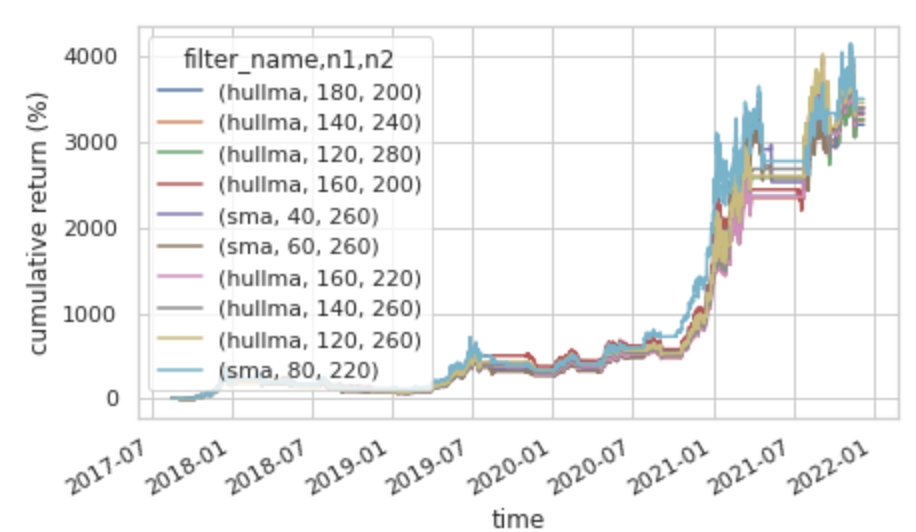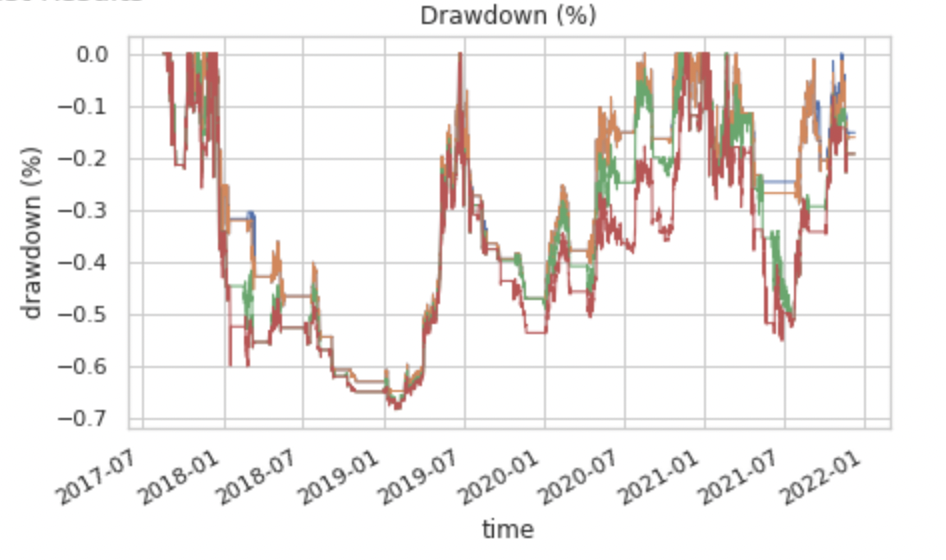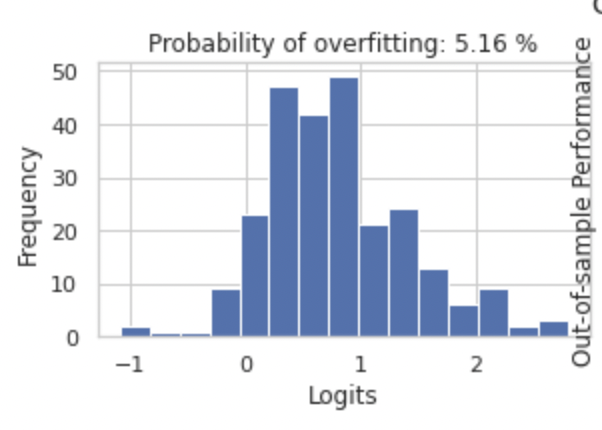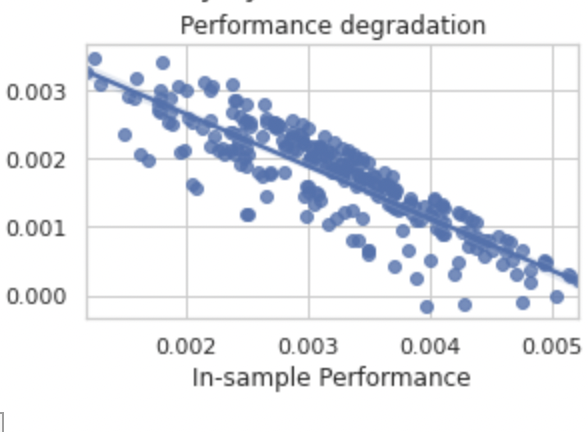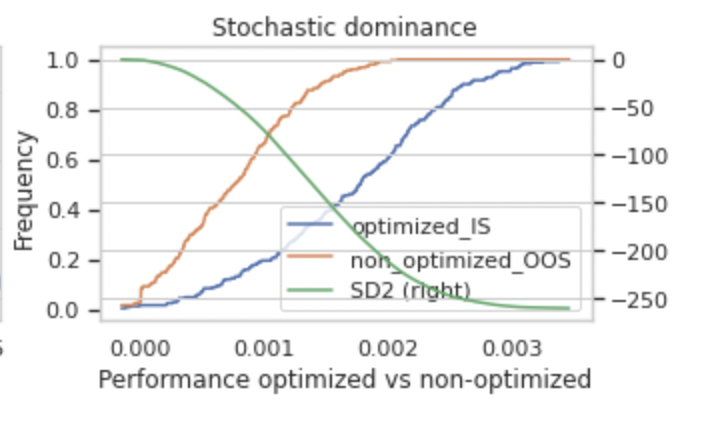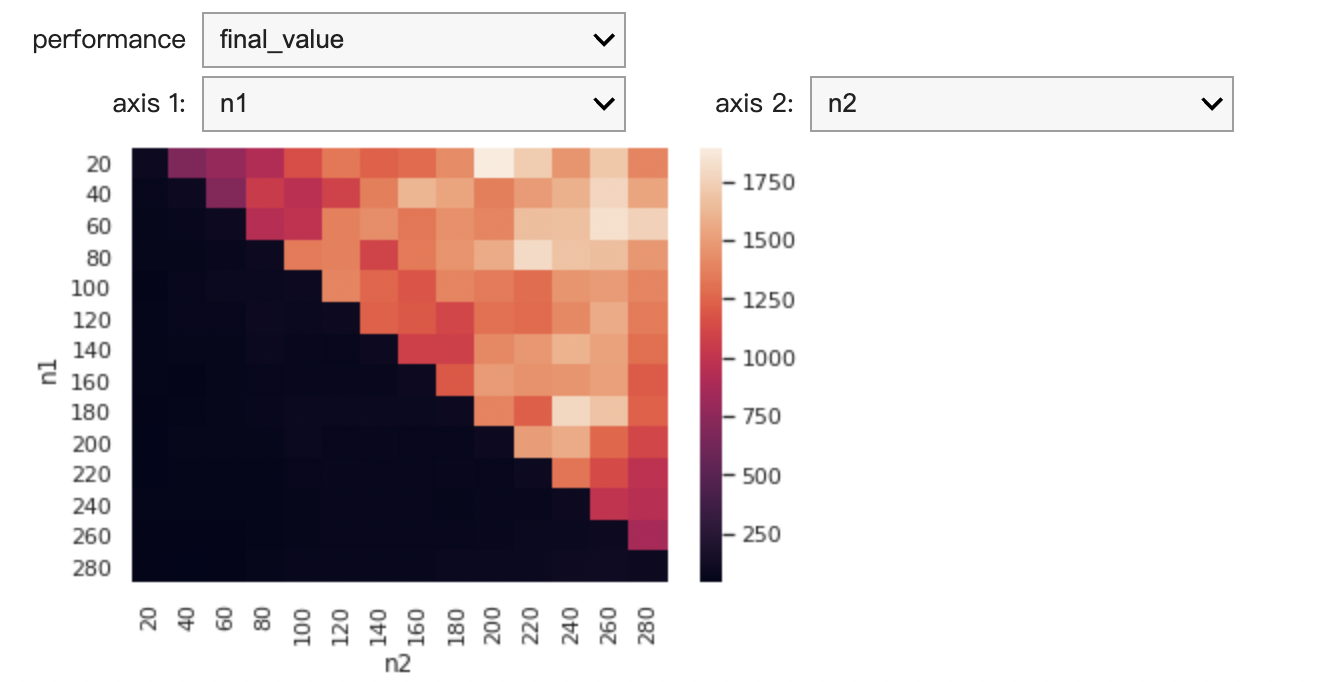# 第二章：2 實戰趨勢策略最佳化

### a. 策略打包

from finlab_crypto import Strategy

@Strategy()
def trend_strategy(ohlcv):


from finlab_crypto import Strategy

@Strategy()
def trend_strategy(ohlcv):

filter_name = 'wma'

close = ohlcv.close

sma20 = indicators.trends[filter_name](close, 20)

sma60 = indicators.trends[filter_name](close, 60)

entries = (sma20 > sma60) & (sma20.shift() < sma60.shift())

exits = (sma20 < sma60) & (sma20.shift() > sma60.shift())

return entries, exits


trend_strategy.backtest(ohlcv, freq = '4h', plot = True)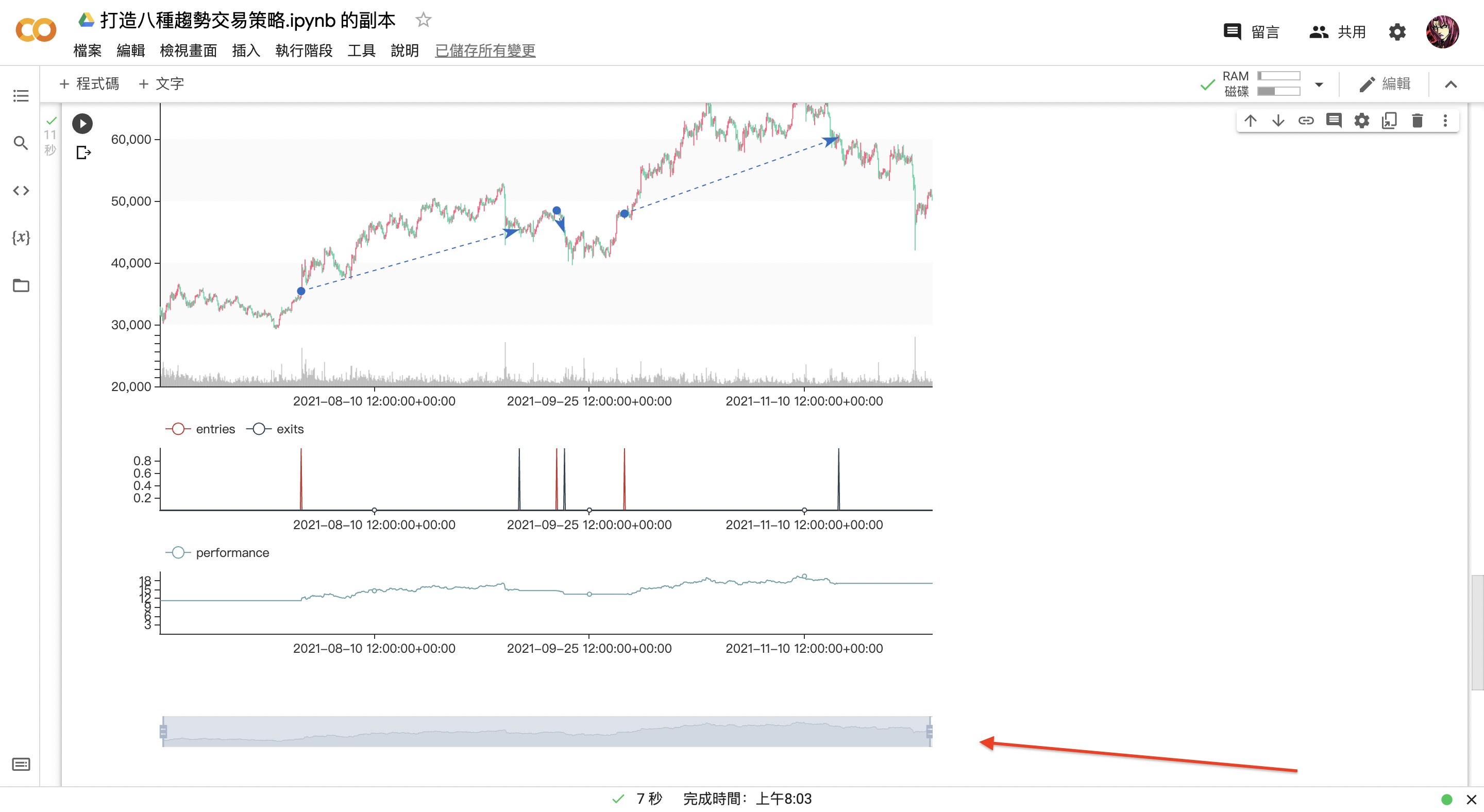### b. 策略最佳化

@Strategy(filter_name = 'sma', n1 = 20, n2 = 60)


from finlab_crypto import Strategy

@Strategy(filter_name = 'sma', n1 = 20, n2 = 60)
def trend_strategy(ohlcv):

filter_name = trend_strategy.filter_name

n1 = trend_strategy.n1

n2 = trend_strategy.n2

close = ohlcv.close

sma20 = indicators.trends[filter_name](close, n1)

sma60 = indicators.trends[filter_name](close, n2)

entries = (sma20 > sma60) & (sma20.shift() < sma60.shift())

exits = (sma20 < sma60) & (sma20.shift() > sma60.shift())

return entries, exits

trend_strategy.backtest(ohlcv, freq = '4h', plot = True)


list(indicators.trends.keys())


variables = {'filter_name': ,'n1':, 'n2': }


variables = {'filter_name': list(indicators.trends.keys()),'n1':, 'n2': }


from finlab_crypto import Strategy

@Strategy(filter_name = 'sma', n1 = 20, n2 = 60)
def trend_strategy(ohlcv):

filter_name = trend_strategy.filter_name

n1 = trend_strategy.n1

n2 = trend_strategy.n2

close = ohlcv.close

sma20 = indicators.trends[filter_name](close, n1)

sma60 = indicators.trends[filter_name](close, n2)

entries = (sma20 > sma60) & (sma20.shift() < sma60.shift())

exits = (sma20 < sma60) & (sma20.shift() > sma60.shift())

return entries, exits

import numpy as np

variables = {
'filter_name': list(indicators.trends.keys()),

'n1': np.arange(20, 300, 20),

'n2': np.arange(20, 300, 20)
}

trend_strategy.backtest(ohlcv, variables = variables, freq = '4h', plot = True)